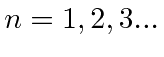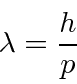# The Problems with Classical Physics

By the late nineteenth century the laws of physics were based on Mechanics and the law of Gravitation from Newton, Maxwell's equations describing Electricity and Magnetism, and on Statistical Mechanics describing the state of large collection of matter. These laws of physics described nature very well under most conditions, however, some measurements of the late 19th and early 20th century could not be understood. The problems with classical physics led to the development of Quantum Mechanics and Special Relativity.

Some of the problems leading to the development of Quantum Mechanics are listed here.

• Black Body Radiation: Classical physics predicted that hot objects would instantly radiate away all their heat into electromagnetic waves. The calculation, which was based on Maxwell's equations and Statistical Mechanics, showed that the radiation rate went to infinity as the EM wavelength went to zero, The Ultraviolet Catastrophe''. Plank solved the problem by postulating that EM energy was emitted in quanta with.

• The Photoelectric Effect: When light was used to knock electrons out of solids, the results were completely different than expected from Maxwell's equations. The measurements were easy to explain (for Einstein) if light is made up of particles with the energies Plank postulated.

• Atoms: After Rutherford found that the positive charge in atoms was concentrated in a very tiny nucleus, classical physics predicted that the atomic electrons orbiting the nucleus would radiate their energy away and spiral into the nucleus. This clearly did not happen. The energy radiated by atoms also came out in quantized amounts in contradiction to the predictions of classical physics. The Bohr Atom postulated an angular momentum quantization rule,for, that gave the right result for hydrogen, but turned out to be wrong since the ground state of hydrogen has zero angular momentum. It took a full understanding of Quantum Mechanics to explain the atomic energy spectra.

• Compton Scattering: When light was scattered off electrons, it behaved just like a particle but changes wave length in the scattering; more evidence for the particle nature of light and Plank's postulate.

• Waves and Particles: In diffraction experiments,light was shown to behave like a wave while in experiments like the Photoelectric effect, light behaved like a particle. More difficult diffraction experiments showed that electrons (as well as the other particles) also behaved like a wave, yet we can only detect an integer number of electrons (or photons).

Quantum Mechanics incorporates a wave-particle duality and explains all of the above phenomena. In doing so, Quantum Mechanics changes our understanding of nature in fundamental ways. While the classical laws of physics are deterministic, QM is probabilistic. We can only predict the probability that a particle will be found in some region of space.

Electromagnetic waves like light are made up of particles we call photons. Einstein, based on Plank's formula, hypothesized that the particles of light had energy proportional to their frequency.The new idea of Quantum Mechanics is that every particle's probability (as a function of position and time) is equal to the square of a probability amplitude function and that these probability amplitudes obey a wave equation. This is much like the case in electromagnetism where the energy density goes like the square of the field and hence the photon probability density goes like the square of the field, yet the field is made up of waves. So probability amplitudes are like the fields we know from electromagnetism in many ways.

DeBroglie assumedfor photons and other particles and used Lorentz invariance (from special relativity) to derive the wavelength for particles like electrons.The rest of wave mechanics was built around these ideas, giving a complete picture that could explain the above measurements and could be tested to very high accuracy, particularly in the hydrogen atom. We will spend several chapters exploring these ideas.

* Example: Assume the photon is a particle with the standard deBroglie wavelength. Use kinematics to derive the wavelength of the scattered photon as a function of angle for Compton Scattering.*

Gasiorowicz Chapter 1

Rohlf Chapters 3,6

Griffiths does not really cover this.

Cohen-Tannoudji et al. Chapter

Subsections
Jim Branson 2013-04-22